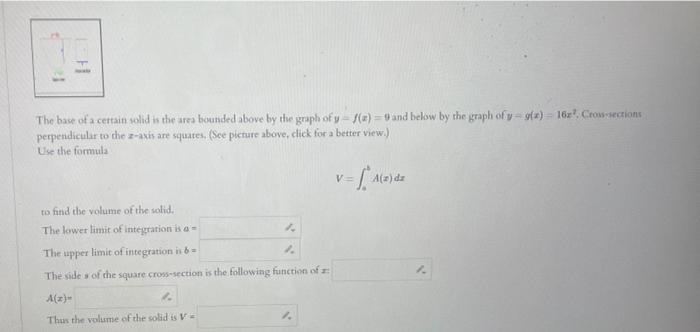# (Solved): The base of a cettain wolid is the area bounded above by the graph of $$y-f(x)=9$$ and below by t ...The base of a cettain wolid is the area bounded above by the graph of $$y-f(x)=9$$ and below by the graph of $$y-g(x)=16 x^{2}$$, Crowsections perpendicular to the $$z$$ - axis are squates. (See picture above, dick for a better view.) Use the formula $V=\int_{0}^{b} A(x) d x$ to find the volume of the solid. The lower limit of integration is $$a=$$ The upper limit of integration in $$b=$$ The side s of the square cross-section is the following function of $$x$$ $A(x)=$ Thas the volume of the solid is $$V=$$

We have an Answer from Expert